##MSIPO技术圈 首页 IT技术 查看内容

2023-07-13

1.排序的概念及其运用

1.1排序的概念

1.2排序运用

1.3常见的七大排序

2.直接插入排序

2.1基本思想

2.2直接插入排序

2.3动图助解

2.4直接插入排序源码

2.5直接插入排序的特性总结

3.希尔排序( 缩小增量排序 )

3.1希尔排序概念及思想

3.2希尔排序图解

3.3希尔排序源码

3.4希尔排序的两种预排序图解

3.5希尔排序的特性总结

# 1.排序的概念及其运用

## 1.2排序运用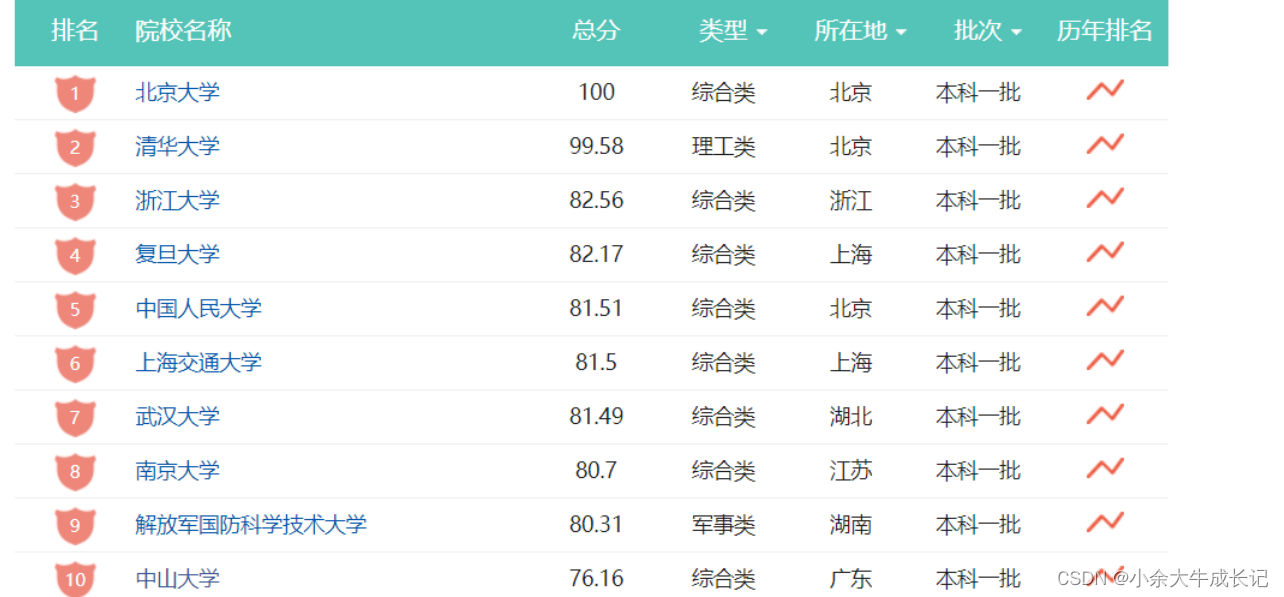## 1.3常见的七大排序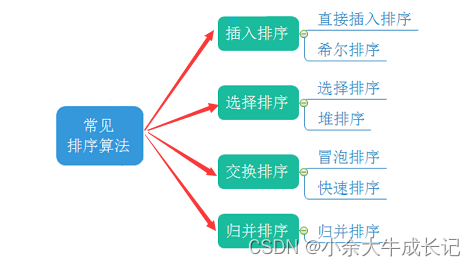# 2.直接插入排序

## 2.1基本思想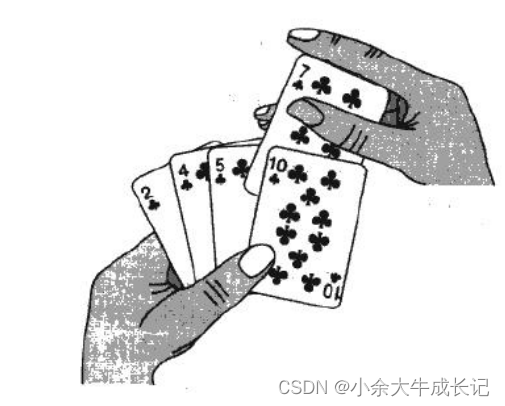## 2.4直接插入排序源码

``````void InsertSort(int* a, int n)
{
for (int i = 0; i < n - 1; ++i)
{
// [0,end]有序，把end+1位置的值插入，保持有序
int end = i;
int tmp = a[end + 1];//插入的值和前面[0,end]的值进行比较
while (end >= 0)
{
if (tmp < a[end])//插入的值小，就往前挪一下
{
a[end + 1] = a[end];
--end;
}
else//到这里就是数组中的值<插入的值 || 整个[0,end]的值都>插入的值
{
break;
}
}
a[end + 1] = tmp;//放入插入值
}
}
``````

## 2.5直接插入排序的特性总结

1. 元素集合越接近有序，直接插入排序算法的时间效率越高
①最优：顺序有序/接近顺序有序 —O(N)
2. 时间复杂度
②最坏：逆序—O(N^2)
3. 空间复杂度：O(1)，它是一种稳定的排序算法
4. 稳定性：稳定

# 3.希尔排序( 缩小增量排序 )

【希尔排序】是对上面【直接插入排序】的最坏情况进行优化。

## 3.2希尔排序图解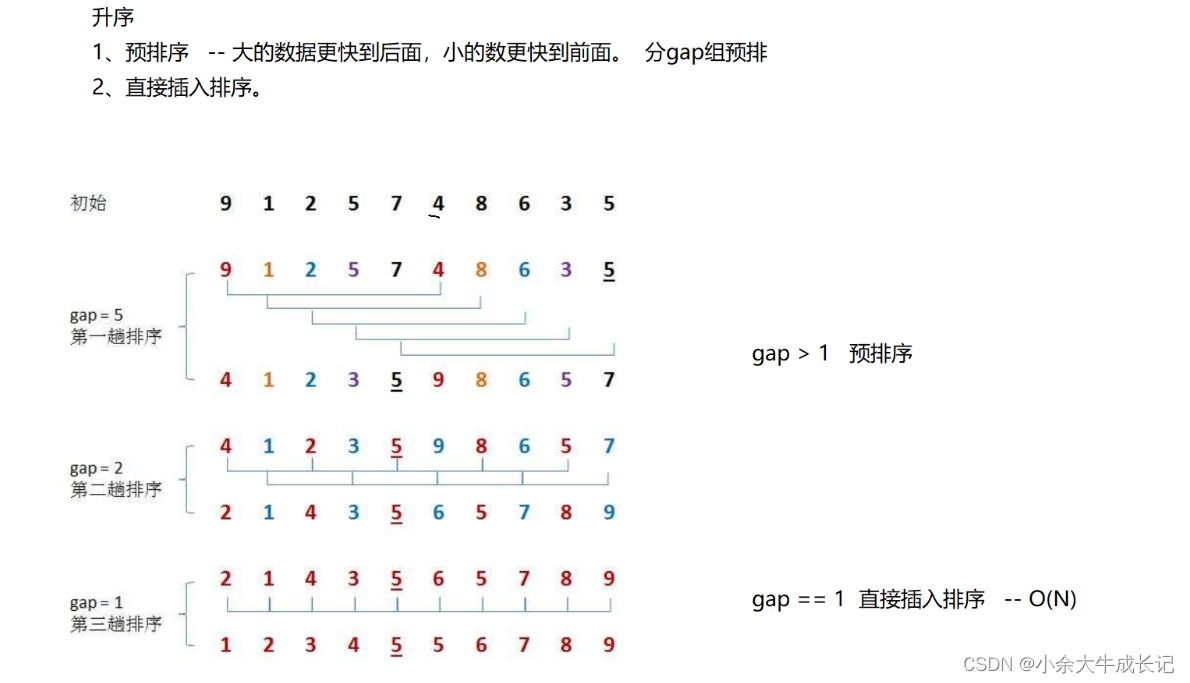【希尔排序】And【直接插入排序】单趟排序的区别## 3.3希尔排序源码

``````void ShellSort(int* a, int n)
{
//方法一：双层循环（不太推荐）
/*int gap = 3;*/

/*for (int j = 0; j < gap; ++j)
{
for (int i = j; i < n - gap; i += gap)
{
int end = i;
int tmp = a[end + gap];
while (end >= 0)
{
if (tmp < a[end])
{
a[end + gap] = a[end];
end -= gap;
}
else
{
break;
}
}
a[end + gap] = tmp;
}
}*/
///

//方法二：单层for循环（推荐）
// gap > 1时是预排序
// gap 最后一次等于1，是直接插入排序

int gap = n;
while (gap > 1)
{
gap = gap / 3 + 1;//保证最后一次gap=1，就是【直接插入排序】了。

for (int i = 0; i < n - gap; ++i)
{
int end = i;
int tmp = a[end + gap];
while (end >= 0)
{
if (tmp < a[end])
{
a[end + gap] = a[end];
end -= gap;
}
else
{
break;
}
}
a[end + gap] = tmp;
}
}
}
``````

## 3.4希尔排序的两种预排序图解

（红色）——9 6 4 1

（蓝色）——8 5 3

（紫色）——7 5 2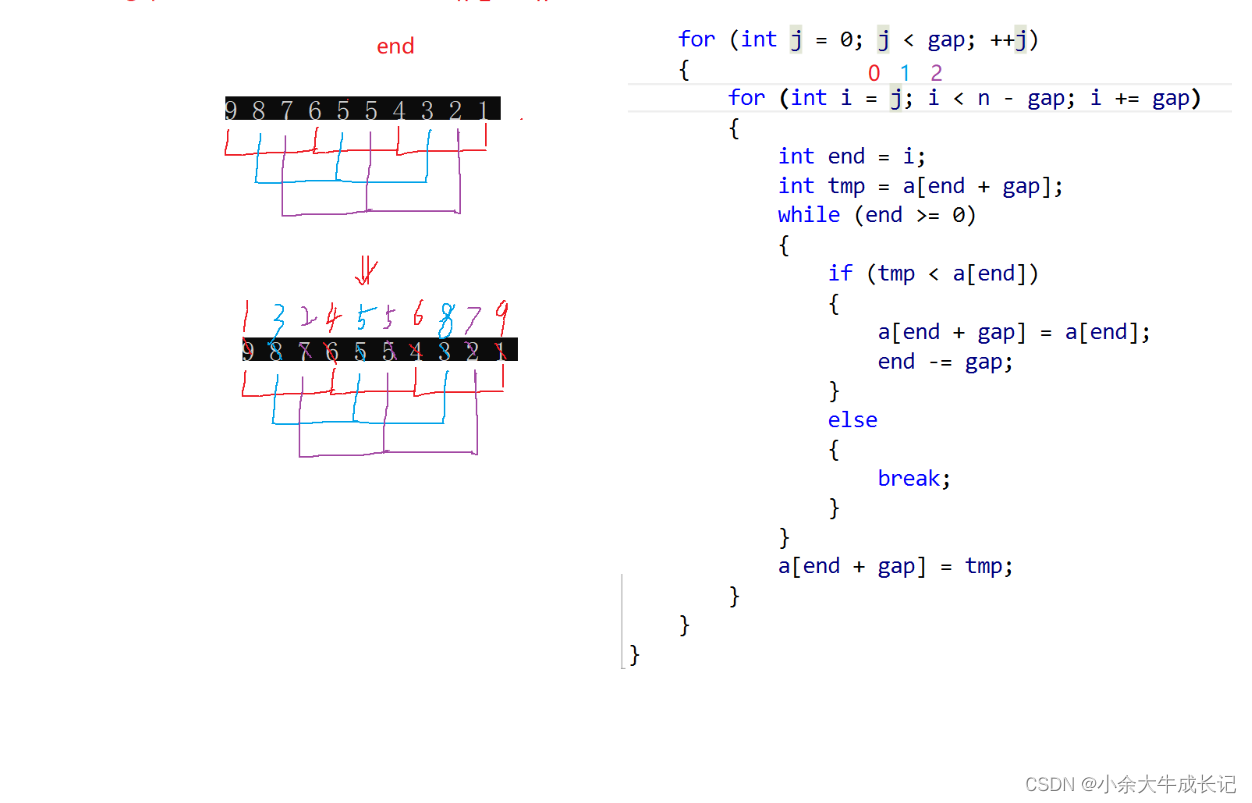方法二【单层for循环控制】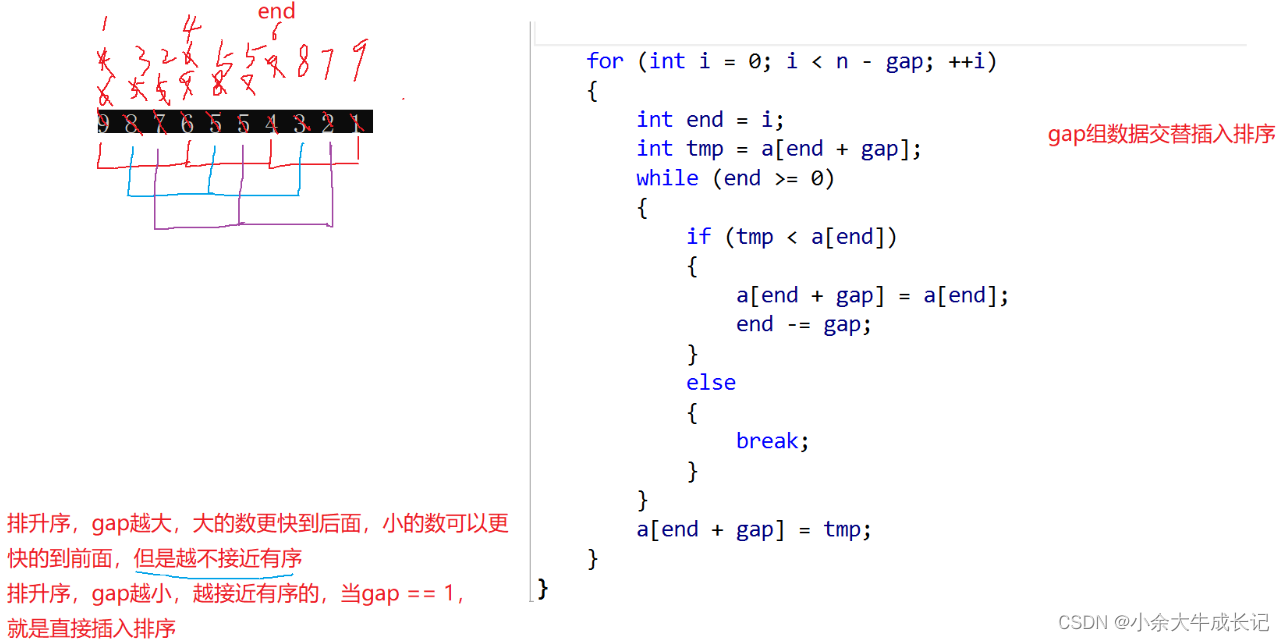## 3.5希尔排序的特性总结

1. 希尔排序是对直接插入排序的优化。
2. gap > 1 时都是预排序，目的是让数组更接近于有序。当 gap == 1 时，数组已经接近有序的了，这样就 会很快。这样整体而言，可以达到优化的效果。我们实现后可以进行性能测试的对比。
3. 希尔排序的时间复杂度不好计算，因为 gap 的取值方法很多，导致很难去计算，因此在好些树中给出的。
4.希尔排序的时间复杂度都不固定：这边给个大概的平均时间复杂度—O(N^1.3)